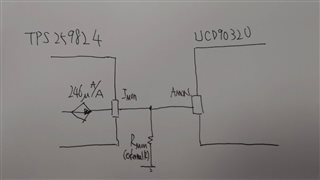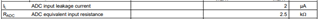If you have a related question, please click the "Ask a related question" button in the top right corner. The newly created question will be automatically linked to this question.

# UCD90320: IMON of TPS259824 connected to AMON pin

Part Number: UCD90320

Hi Yihe :

We want to use IMON of TPS259824 and connect it to AMON of UCD90320 to monitor current consumption.

Since IMON is a current output pin of TPS259824, so I need to check some items with you.-

1. Does RADC means resistance of AMON pin to GND?

Could you help provide typical and minimum value of it to us?-

2. If IL (ADC input leakage current) = max : 2uA and RADC = max : 2.5k ohm

(1) So there will be a Vmax = 5mV on AMON pin to GND => Is it correct?

(2) If RMON is 2.21k ohm, Iout = 6A so there's 6*246uA/A = 1.476mA flow out of IMON pin

a. VAMON(max) will be 1.476mA * (2.21k//2.5k) = 1.731V

b. Or current flow through RMON, min. value is 1.476mA - 2uA = 1.474mA.

So VAMON(min) will be 1.474mA * 2.21k ohm = 3.258V?